Electric Fields Physics Analogy The electric field is

• Slides: 22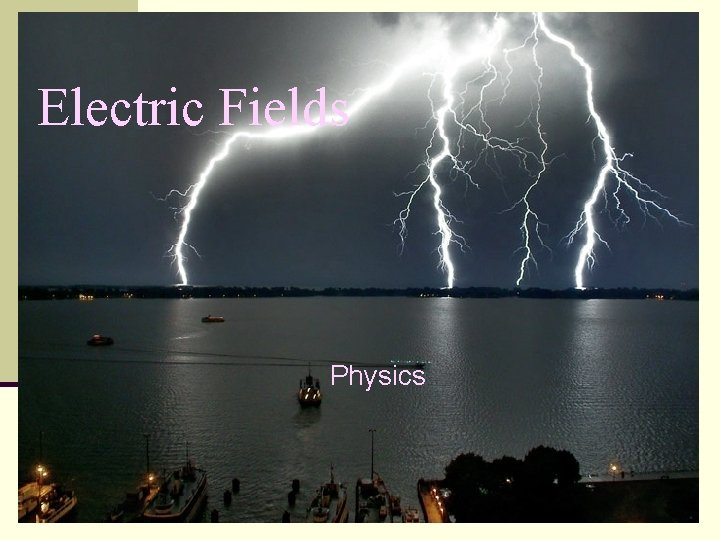Electric Fields Physics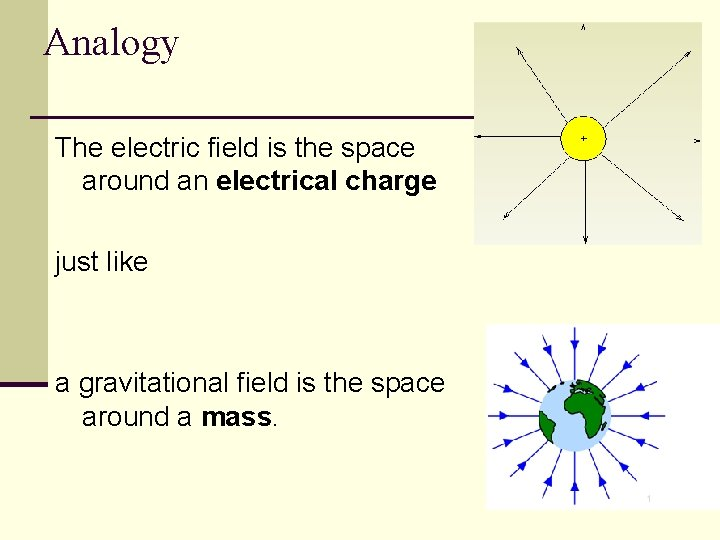Analogy The electric field is the space around an electrical charge just like a gravitational field is the space around a mass.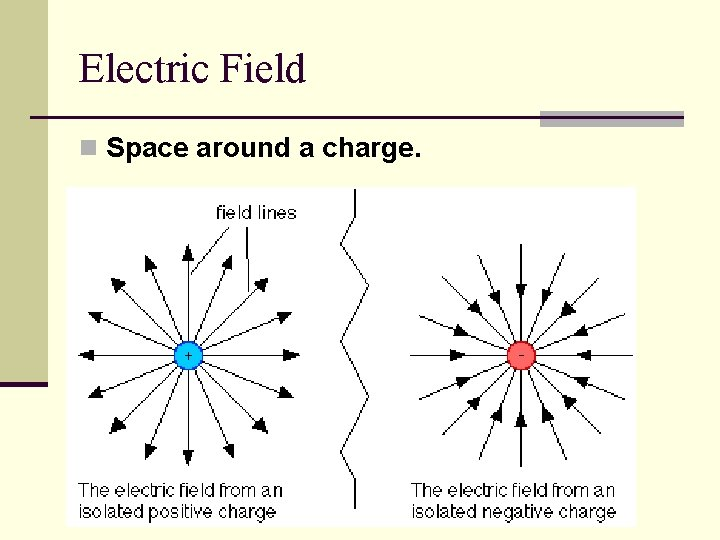Electric Field n Space around a charge.What is the difference?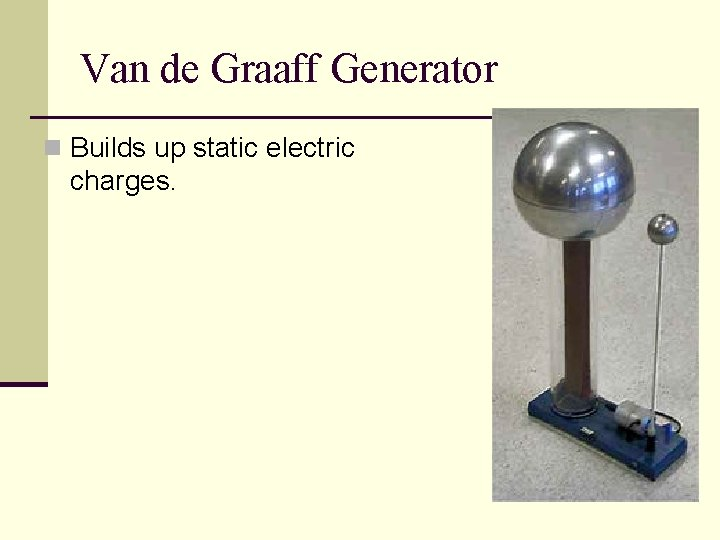Van de Graaff Generator n Builds up static electric charges.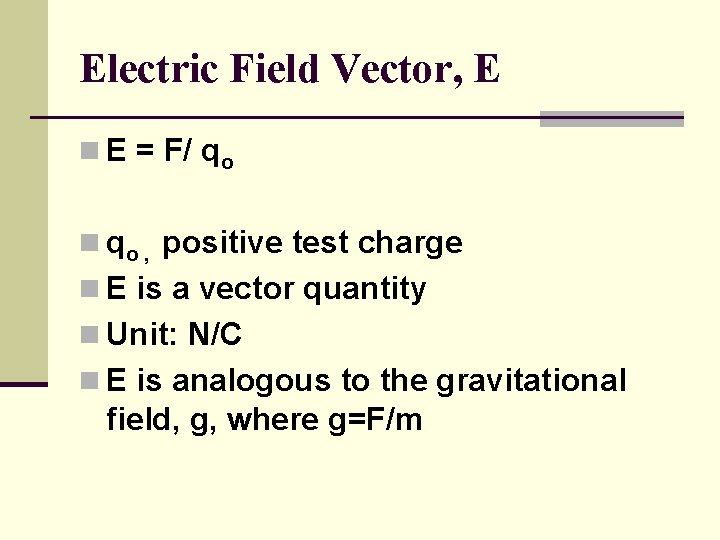Electric Field Vector, E n E = F/ qo n qo , positive test charge n E is a vector quantity n Unit: N/C n E is analogous to the gravitational field, g, where g=F/m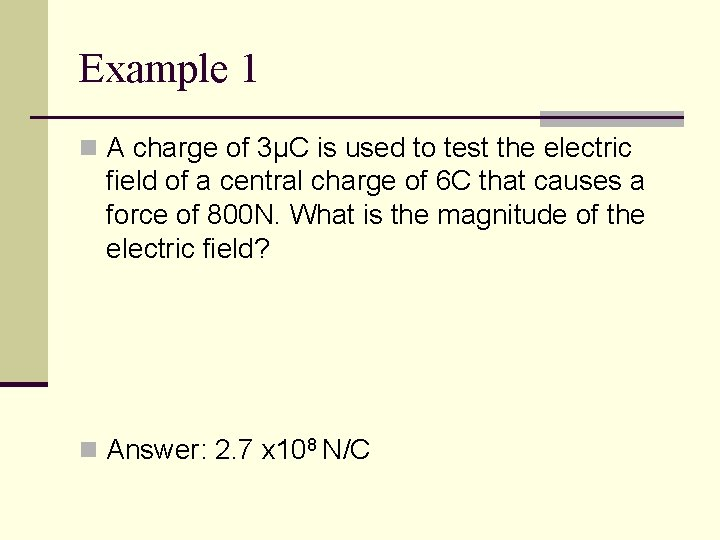Example 1 n A charge of 3µC is used to test the electric field of a central charge of 6 C that causes a force of 800 N. What is the magnitude of the electric field? n Answer: 2. 7 x 108 N/C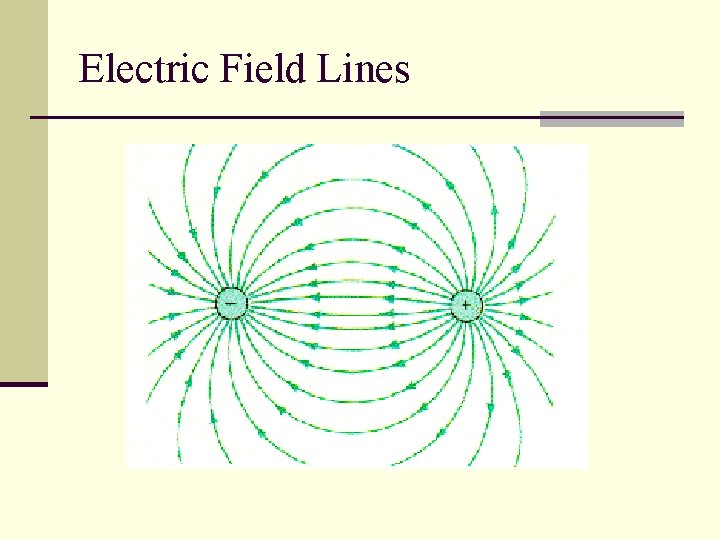Electric Field Lines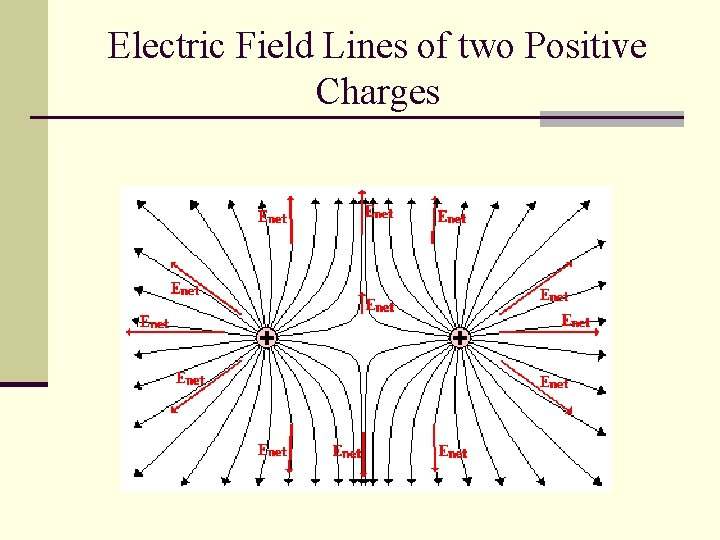Electric Field Lines of two Positive ChargesElectric Field Lines n Lines that indicate the strength and direction of the electric field. n The more dense the lines, the stronger the field.Example 2 n A positive test charge is placed between an electron e and a proton p. Which way will the test charge move? e- p+ +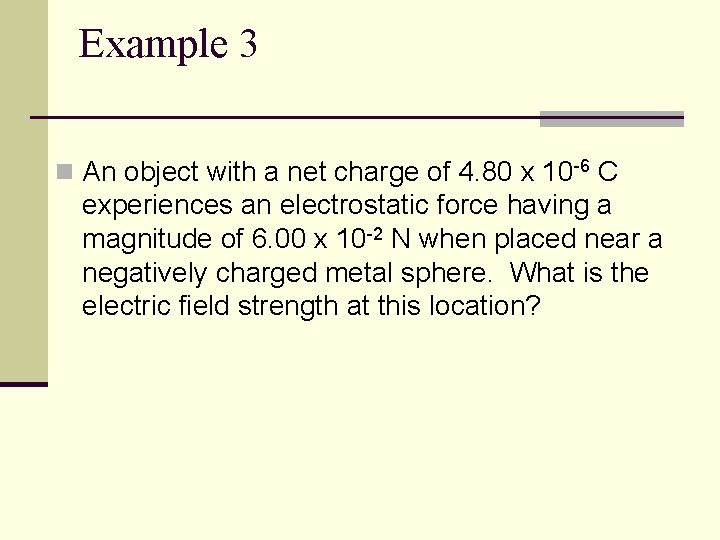Example 3 n An object with a net charge of 4. 80 x 10 -6 C experiences an electrostatic force having a magnitude of 6. 00 x 10 -2 N when placed near a negatively charged metal sphere. What is the electric field strength at this location?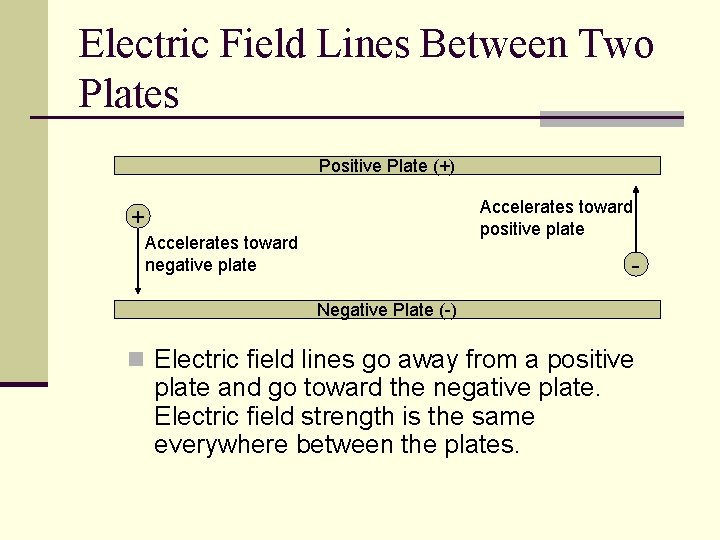Electric Field Lines Between Two Plates Positive Plate (+) Accelerates toward positive plate + Accelerates toward negative plate Negative Plate (-) n Electric field lines go away from a positive plate and go toward the negative plate. Electric field strength is the same everywhere between the plates.Final Thought… +++++++++++++++++ p+ n e- - - - - - - - n A proton, neutron and electron are located between two oppositely charged plates, the magnitude of acceleration will be greatest for the n Proton n Neutron n electron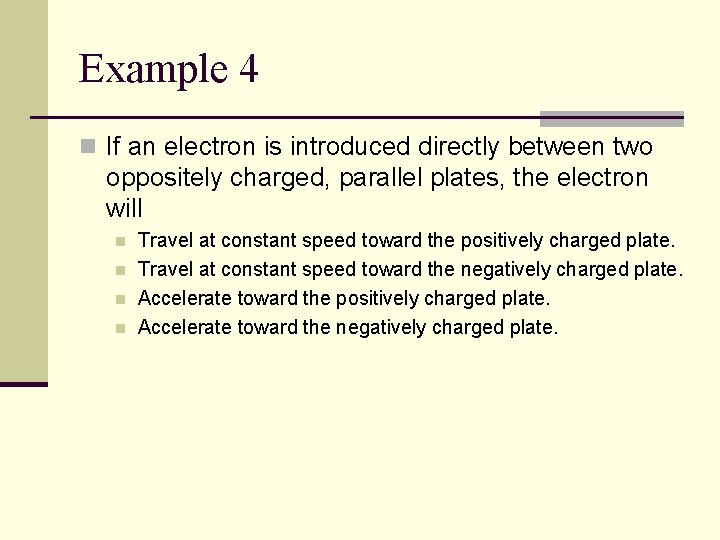Example 4 n If an electron is introduced directly between two oppositely charged, parallel plates, the electron will n n Travel at constant speed toward the positively charged plate. Travel at constant speed toward the negatively charged plate. Accelerate toward the positively charged plate. Accelerate toward the negatively charged plate.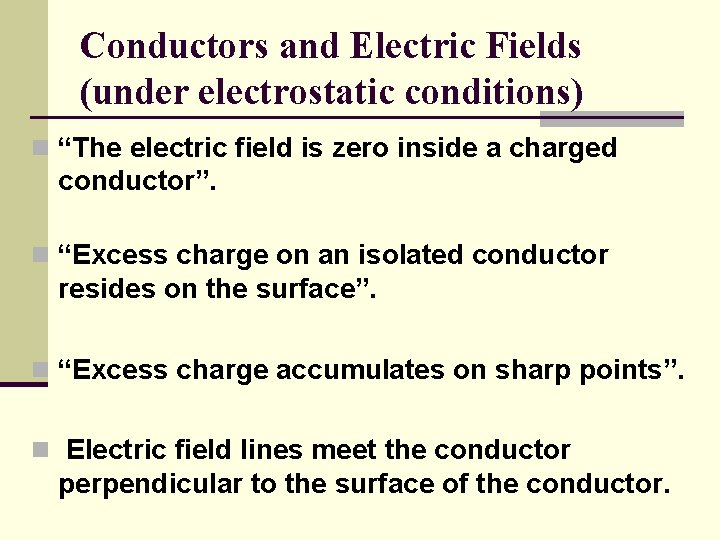Conductors and Electric Fields (under electrostatic conditions) n “The electric field is zero inside a charged conductor”. n “Excess charge on an isolated conductor resides on the surface”. n “Excess charge accumulates on sharp points”. n Electric field lines meet the conductor perpendicular to the surface of the conductor.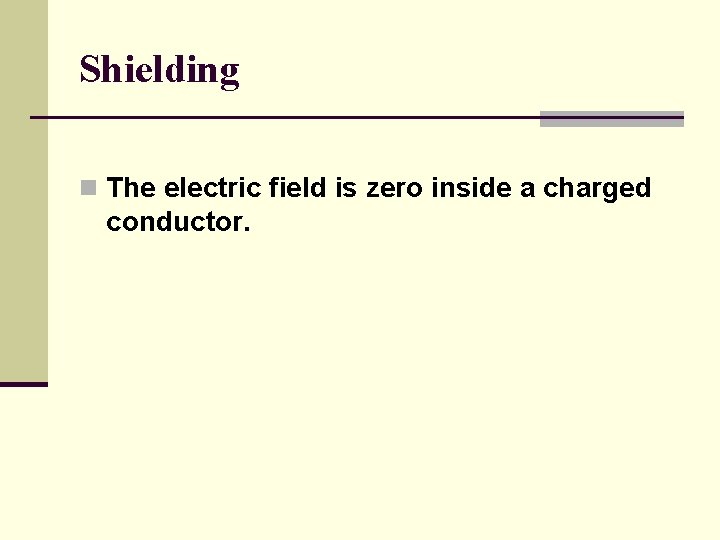Shielding n The electric field is zero inside a charged conductor.Where are you safe during a thunderstorm? A) In a car B) Outdoors orWhy can you not get radio reception in a tunnel or in a steel bridge?Which field is stronger? n. A BExample 5 n A test charge of +3µC is located 5 m to the east of a -4µC charge. n A) Find the electric force felt by the test charge. n B) Find the electric field at that location. n Answer: 4. 32 x 10 -3 N, 1. 44 x 103 N/C along the –x axis.Example 6 n If a test charge is moved to a location three times as far as its original location, how does the electric field change?